### Home > MC2 > Chapter 5 > Lesson 5.3.1 > Problem5-92

5-92.
1. Copy each problem, then find the sum, difference, product, or quotient. Remember to show all your steps. Homework Help ✎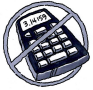1. 23.6 + 12

2. 16.5 + 52.43

3. 46.21 – 31.2

4. 27.5 – 13.11

5. 4.5(6)

6. 55 ÷ 2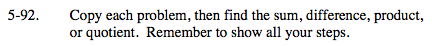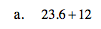To add numbers with decimals remember to line up the decimal points.

35.6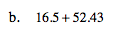See part (a).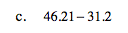To find the difference between numbers with decimals remember to line up the decimal points.

15.01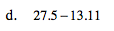See part (c).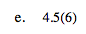Remember, when you multiply numbers with decimals, you do not need to line up the decimal points.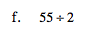Remember to add a decimal and zero. 2 doesn't fit evenly into 55.